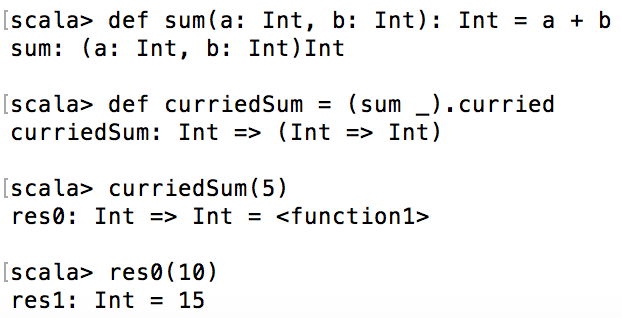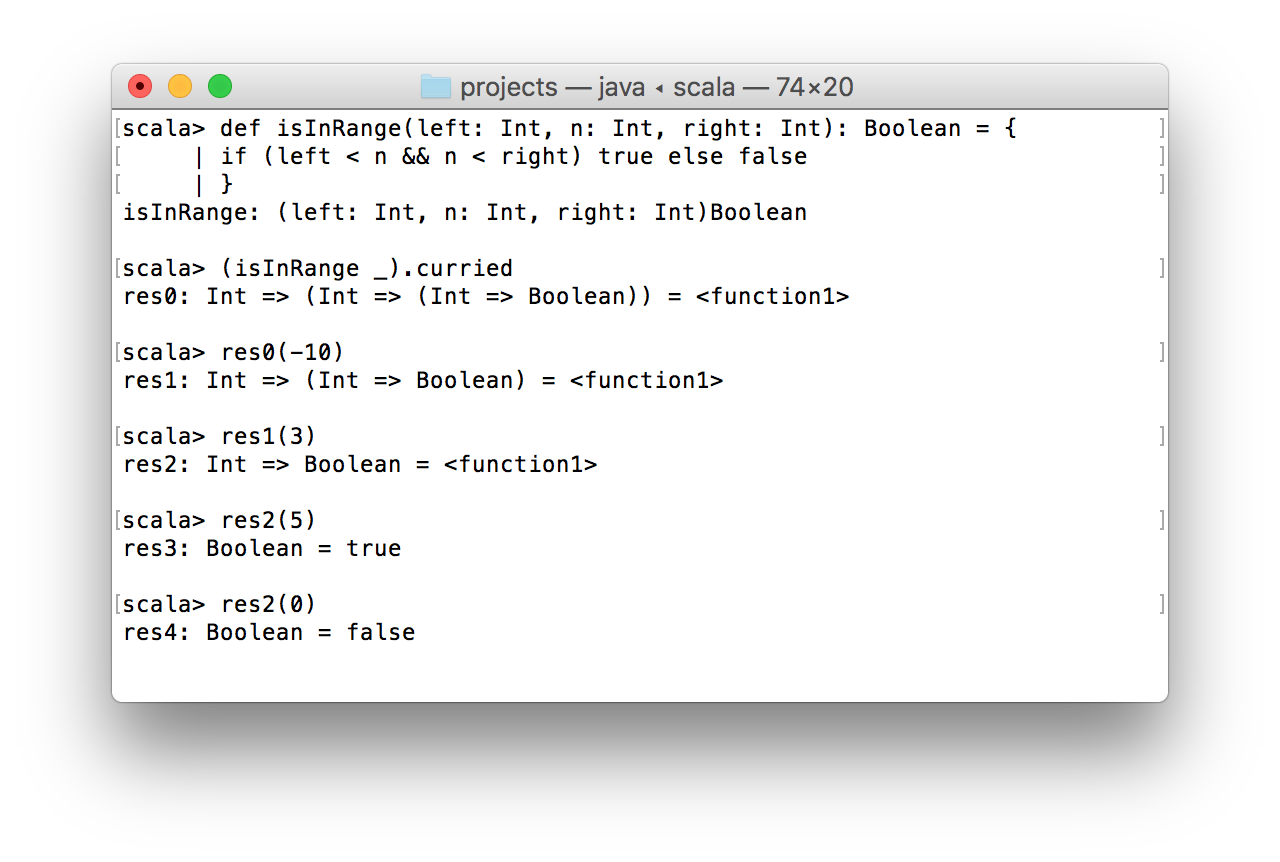In this article I want to show a real difference between curried functions and partially applied functions in Scala. This question is pretty common for those developers who started learning Scala without previous experience in functional programming. Moreover, this blog post may be useful, even for experienced Scala developers, because based on my experience I have had incorrect understanding of the difference between the curried functions and partially applied functions.

So here is the most classic example of misunderstanding in question of currying and partially applied functions. I wrote that article ~9 months ago. Since that time I dived more deeper in Scala and figured out what is a curried function and what is a partially applied function.

## Definitions

Before demonstration of code examples, it would be rational to get acquainted with the definitions. For most people it is enough in order to understand what is the difference.

Currying – decomposition of function with multiple arguments into a chain of single-argument functions.

Notice, that Scala allows to pass a function as an argument to another function.

Partial application of function – pass to function less arguments than it has in its declaration. Scala does not throw an exception when you provide less arguments to function, it simply applies them and return a new function with rest of arguments which need to be passed.

In general, you can stop reading this article if everything is clear. Otherwise I recommend to look at some examples for each of function types.

## Currying examples

Let’s start from the most trivial example of curried function:

```def sum(a: Int, b: Int): Int = a + b
//(Int, Int) => Int

def curriedSum = (sum _).curried
//Int => (Int => Int)

curriedSum(5)
//Int => Int

res0(10)
//Int = 15
```

What happened in the code snippet above? At first I declared function `sum`. It has two arguments of `Int` type.Its returning type is `Int` as well.
Then I create a curried version of the `sum` function. The `curriedSum` function accepts single argument of `Int` type and returns a function of `Int => Int` type.
When I pass 5 into the `curriedSum` I receive back a new function `res0: Int => Int` (this sample was performed in REPL).
Finally, invoking `res0` with any `Int` parameter we will add this parameter to 5.By invoking a `curried` method on any function of type `FunctionN` where N > 1, we receive a curried version of the function.

Here is another example of currying:

```def isInRange(left: Int, n: Int, right: Int): Boolean = {
if (left < n && n < right) true else false
}

(isInRange _).curried
//Int => (Int => (Int => Boolean))
```

The logic of `isInRange` function is pretty simple: if argument `n` more than `left` but less than `right`, the function returns `true`, otherwise `false`.

After we made a currying version of `isInRange`, we can perform following operations with it:On this screenshot you can see how we pass one by one arguments and as a result we see two boolean results in `res3` and `res4`.

## Partial application examples

Now let’s see how partially applied functions look like on practice. I’m going to use the `isInRange` function from the previous section.

```def isInRange(left: Int, n: Int, right: Int): Boolean = {
if (left < n && n < right) true else false
}

def is5InRange = isInRange(_: Int, 5, _: Int)
//(Int, Int) => Boolean

is5InRange(0, 8)
//true

def between0and10 = isInRange(0, _: Int, 10)
//Int => Boolean

between0and10(5)
//true

between0and10(100)
//false
```

So what happened in the code snippet above? I used `isInRange` function as a base for 2 new functions: `is5InRange` and `between0and10`. Notice, that for missed parameters you can use underscore.

Partial application is extremely helpful when you want to create a set of different functions on top of one particular function. In the same way, as I create `between0and10`, it’s easy to create `between50and75` for instance.

## Summary

So after the theory and practice part, I guess you understood how curried functions differs from partially applied functions. Once again: currying – transformation of a function with multiple arguments into a chain of single-argument functions, partially applied function – function which was called with less number of arguments.

### About The Author

##### Alex Fruzenshtein

Mathematician, programmer, wrestler, last action hero... Java / Scala architect, trainer, entrepreneur, author of this blog